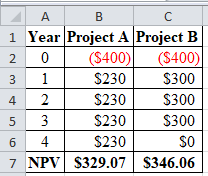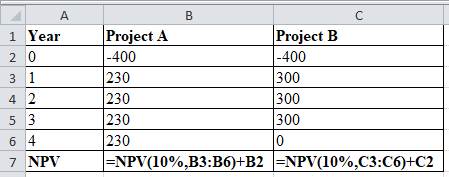# The following are the cash flows of two projects: YearProject AProject B0\$(400)\$(400)1 230  300 2 230  300 3 230  300 4 230      a. Calculate the NPV for both projects if the discount rate is 10%. (Do not round intermediate calculations. Round your answers to 2 decimal places.)     b. Suppose that you can choose only one of these projects. Which would you choose?  Project BProject ANeither

Question
42 views

The following are the cash flows of two projects:

 Year Project A Project B 0 \$ (400 ) \$ (400 ) 1 230 300 2 230 300 3 230 300 4 230

a. Calculate the NPV for both projects if the discount rate is 10%. (Do not round intermediate calculations. Round your answers to 2 decimal places.)

b. Suppose that you can choose only one of these projects. Which would you choose?

• Project B
• Project A
• Neither

check_circle

star
star
star
star
star
1 Rating
Step 1

a)

NPV for Project A and project B is \$329.07 and \$346.06.

Computation of NPV:Step 2

Excel workings:...

### Want to see the full answer?

See Solution

#### Want to see this answer and more?

Solutions are written by subject experts who are available 24/7. Questions are typically answered within 1 hour.*

See Solution
*Response times may vary by subject and question.
Tagged in